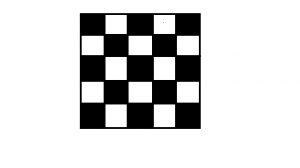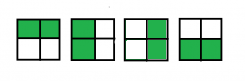Skip to content
Related Articles
Ways to place K bishops on an N×N chessboard so that no two attack
• Difficulty Level : Hard
• Last Updated : 06 May, 2021

Given two integers N and K, the task is to find the number of ways to place K bishops on an N × N chessboard so that no two bishops attack each other.

Here is an example for a 5×5 chessboard.Examples:

Input: N = 2, K = 2
Output:
The different ways to place 2 bishops in a 2 * 2 chessboard are :Input: N = 4, K = 3
Output: 232

Approach: This problem can be solved using dynamic programming

• Let dp[i][j] denote the number of ways to place j bishops on diagonals with indices up to i which have the same color as diagonal i. Then i = 1…2N-1 and j = 0…K.
• We can calculate dp[i][j] using only values of dp[i-2] (we subtract 2 because we only consider diagonals of the same color as i). There are two ways to get dp[i][j]. Either we place all j bishops on previous diagonals: then there are dp[i-2][j] ways to achieve this. Or we place one bishop on diagonal i and j-1 bishops on previous diagonals. The number of ways to do this equals the number of squares in diagonal i – (j – 1), because each of j-1 bishops placed on previous diagonals will block one square on the current diagonal.
• The base case is simple: dp[i] = 1, dp = 1.
• Once we have calculated all values of dp[i][j], the answer can be obtained as follows: consider all possible numbers of bishops placed on black diagonals i=0…K, with corresponding numbers of bishops on white diagonals K-i. The bishops placed on black and white diagonals never attack each other, so the placements can be done independently. The index of the last black diagonal is 2N-1, the last white one is 2N-2. For each i we add dp[2N-1][i] * dp[2N-2][K-i] to the answer.

Below is the implementation of the above approach:

## C++

 `// CPP implementation of the approach``#include``using` `namespace` `std;` `// returns the number of squares in diagonal i``int` `squares(``int` `i)``{``    ` `    ``if` `((i & 1) == 1)``        ``return` `i / 4 * 2 + 1;``    ``else``        ``return` `(i - 1) / 4 * 2 + 2;``}` `// returns the number of ways to fill a``// n * n chessboard with k bishops so``// that no two bishops attack each other.``long` `bishop_placements(``int` `n, ``int` `k)``{``    ``// return 0 if the number of valid places to be``    ``// filled is less than the number of bishops``    ``if` `(k > 2 * n - 1)``        ``return` `0;` `    ``// dp table to store the values``    ``long` `dp[n * 2][k + 1];` `    ``// Setting the base conditions``    ``for``(``int` `i = 0; i < n * 2; i++)``    ``{``        ``for``(``int` `j = 0; j < k + 1; j++)``        ``{``            ``dp[i][j] = 0;``        ``}``    ` `    ``}``    ``for` `(``int` `i = 0; i < n * 2; i++)``        ``dp[i] = 1;``    ``dp = 1;` `    ``// calculate the required number of ways``    ``for` `(``int` `i = 2; i < n * 2; i++)``    ``{``        ``for` `(``int` `j = 1; j <= k; j++)``        ``{``            ``dp[i][j] = dp[i - 2][j]``                    ``+ dp[i - 2][j - 1] * (squares(i) - j + 1);` `        ``}``    ``}` `    ``// stores the answer``    ``long` `ans = 0;``    ``for` `(``int` `i = 0; i <= k; i++)``    ``{``        ``ans += dp[n * 2 - 1][i] * dp[n * 2 - 2][k - i];``    ``}` `    ``return` `ans;``}` `// Driver code``int` `main()``{``    ``int` `n = 2;``    ``int` `k = 2;``    ``long` `ans = bishop_placements(n, k);``    ``cout << (ans);``}` `// This code is contributed by Rajput-Ji`

## Java

 `// Java implementation of the approach` `class` `GFG {` `    ``// returns the number of squares in diagonal i``    ``static` `int` `squares(``int` `i)``    ``{``        ``if` `((i & ``1``) == ``1``)``            ``return` `i / ``4` `* ``2` `+ ``1``;``        ``else``            ``return` `(i - ``1``) / ``4` `* ``2` `+ ``2``;``    ``}` `    ``// returns the number of ways to fill a``    ``// n * n chessboard with k bishops so``    ``// that no two bishops attack each other.``    ``static` `long` `bishop_placements(``int` `n, ``int` `k)``    ``{``        ``// return 0 if the number of valid places to be``        ``// filled is less than the number of bishops``        ``if` `(k > ``2` `* n - ``1``)``            ``return` `0``;` `        ``// dp table to store the values``        ``long``[][] dp = ``new` `long``[n * ``2``][k + ``1``];` `        ``// Setting the base conditions``        ``for` `(``int` `i = ``0``; i < n * ``2``; i++)``            ``dp[i][``0``] = ``1``;``        ``dp[``1``][``1``] = ``1``;` `        ``// calculate the required number of ways``        ``for` `(``int` `i = ``2``; i < n * ``2``; i++) {``            ``for` `(``int` `j = ``1``; j <= k; j++)``                ``dp[i][j]``                    ``= dp[i - ``2``][j]``                        ``+ dp[i - ``2``][j - ``1``] * (squares(i) - j + ``1``);``        ``}` `        ``// stores the answer``        ``long` `ans = ``0``;``        ``for` `(``int` `i = ``0``; i <= k; i++) {``            ``ans += dp[n * ``2` `- ``1``][i] * dp[n * ``2` `- ``2``][k - i];``        ``}` `        ``return` `ans;``    ``}` `    ``// Driver code``    ``public` `static` `void` `main(String[] args)``    ``{``        ``int` `n = ``2``;``        ``int` `k = ``2``;``        ``long` `ans = bishop_placements(n, k);``        ``System.out.println(ans);``    ``}``}`

## Python3

 `# Python 3 implementation of the approach` `# returns the number of squares in``# diagonal i``def` `squares(i):``    ``if` `((i & ``1``) ``=``=` `1``):``        ``return` `int``(i ``/` `4``) ``*` `2` `+` `1``    ``else``:``        ``return` `int``((i ``-` `1``) ``/` `4``) ``*` `2` `+` `2` `# returns the number of ways to fill a``# n * n chessboard with k bishops so``# that no two bishops attack each other.``def` `bishop_placements(n, k):``    ` `    ``# return 0 if the number of valid places``    ``# to be filled is less than the number``    ``# of bishops``    ``if` `(k > ``2` `*` `n ``-` `1``):``        ``return` `0` `    ``# dp table to store the values``    ``dp ``=` `[[``0` `for` `i ``in` `range``(k ``+` `1``)]``             ``for` `i ``in` `range``(n ``*` `2``)]` `    ``# Setting the base conditions``    ``for` `i ``in` `range``(n ``*` `2``):``        ``dp[i][``0``] ``=` `1``        ` `    ``dp[``1``][``1``] ``=` `1` `    ``# calculate the required number of ways``    ``for` `i ``in` `range``(``2``, n ``*` `2``, ``1``):``        ``for` `j ``in` `range``(``1``, k ``+` `1``, ``1``):``            ``dp[i][j] ``=` `(dp[i ``-` `2``][j] ``+``                        ``dp[i ``-` `2``][j ``-` `1``] ``*``                       ``(squares(i) ``-` `j ``+` `1``))` `    ``# stores the answer``    ``ans ``=` `0``    ``for` `i ``in` `range``(``0``, k ``+` `1``, ``1``):``        ``ans ``+``=` `(dp[n ``*` `2` `-` `1``][i] ``*``                ``dp[n ``*` `2` `-` `2``][k ``-` `i])` `    ``return` `ans` `# Driver code``if` `__name__ ``=``=` `'__main__'``:``    ``n ``=` `2``    ``k ``=` `2``    ``ans ``=` `bishop_placements(n, k)``    ``print``(ans)` `# This code is contributed by``# Sanjit_Prasad`

## C#

 `// C# implementation of the approach``using` `System;` `class` `GFG``{` `// returns the number of squares``// in diagonal i``static` `int` `squares(``int` `i)``{``    ``if` `((i & 1) == 1)``        ``return` `i / 4 * 2 + 1;``    ``else``        ``return` `(i - 1) / 4 * 2 + 2;``}` `// returns the number of ways to fill a``// n * n chessboard with k bishops so``// that no two bishops attack each other.``static` `long` `bishop_placements(``int` `n, ``int` `k)``{``    ``// return 0 if the number of valid``    ``// places to be filled is less than``    ``// the number of bishops``    ``if` `(k > 2 * n - 1)``        ``return` `0;` `    ``// dp table to store the values``    ``long``[,] dp = ``new` `long``[n * 2, k + 1];` `    ``// Setting the base conditions``    ``for` `(``int` `i = 0; i < n * 2; i++)``        ``dp[i, 0] = 1;``    ``dp[1, 1] = 1;` `    ``// calculate the required``    ``// number of ways``    ``for` `(``int` `i = 2; i < n * 2; i++)``    ``{``        ``for` `(``int` `j = 1; j <= k; j++)``            ``dp[i, j] = dp[i - 2, j] +``                       ``dp[i - 2, j - 1] *``                        ``(squares(i) - j + 1);``    ``}` `    ``// stores the answer``    ``long` `ans = 0;``    ``for` `(``int` `i = 0; i <= k; i++)``    ``{``        ``ans += dp[n * 2 - 1, i] *``               ``dp[n * 2 - 2, k - i];``    ``}` `    ``return` `ans;``}` `// Driver code``static` `public` `void` `Main ()``{``    ``int` `n = 2;``    ``int` `k = 2;``    ``long` `ans = bishop_placements(n, k);``    ``Console.WriteLine(ans);``}``}` `// This code is contributed by akt_mit`

## PHP

 ` 2 * ``\$n` `- 1)``        ``return` `0;` `    ``// dp table to store the values``    ``\$dp` `= ``array_fill``(0, ``\$n` `* 2,``          ``array_fill``(0, ``\$k` `+ 1, NULL));` `    ``// Setting the base conditions``    ``for` `(``\$i` `= 0; ``\$i` `< ``\$n` `* 2; ``\$i``++)``        ``\$dp``[``\$i``] = 1;``    ``\$dp`` = 1;` `    ``// calculate the required number of ways``    ``for` `(``\$i` `= 2; ``\$i` `< ``\$n` `* 2; ``\$i``++)``    ``{``        ``for` `(``\$j` `= 1; ``\$j` `<= ``\$k``; ``\$j``++)``            ``\$dp``[``\$i``][``\$j``] = ``\$dp``[``\$i` `- 2][``\$j``] +``                          ``\$dp``[``\$i` `- 2][``\$j` `- 1] *``                             ``(squares(``\$i``) - ``\$j` `+ 1);``    ``}` `    ``// stores the answer``    ``\$ans` `= 0;``    ``for` `(``\$i` `= 0; ``\$i` `<= ``\$k``; ``\$i``++)``    ``{``        ``\$ans` `+= ``\$dp``[``\$n` `* 2 - 1][``\$i``] *``                ``\$dp``[``\$n` `* 2 - 2][``\$k` `- ``\$i``];``    ``}` `    ``return` `\$ans``;``}` `// Driver code``\$n` `= 2;``\$k` `= 2;``\$ans` `= bishop_placements(``\$n``, ``\$k``);``echo` `\$ans``;` `// This code is contributed by ita_c``?>`

## Javascript

 ``
Output:
`4`

Attention reader! Don’t stop learning now. Get hold of all the important DSA concepts with the DSA Self Paced Course at a student-friendly price and become industry ready.  To complete your preparation from learning a language to DS Algo and many more,  please refer Complete Interview Preparation Course.

In case you wish to attend live classes with industry experts, please refer DSA Live Classes

My Personal Notes arrow_drop_up